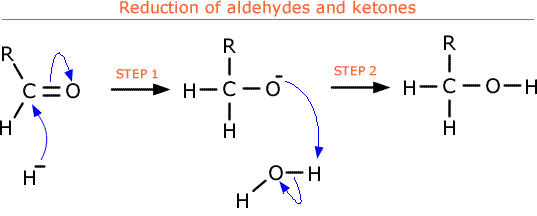# What is the difference between an electrophilic addition and a nucleophilic addition? Is there a rule or easy to understand pattern that can be used to differentiate between the two types?

Oct 23, 2016

Electrophilic addition is where the group being added accepts an electron pair while nucleophilic addition is where the group being added donates an electron pair.

#### Explanation:

One way to do this is to look at the reaction mechanism and see if the electrons move from the group being added or to the group being added.This is electrophilic addition because the $\text{Br}$ atoms accept an electron pair from the $\text{C"-"C}$ double bond.This is nucleophilic addition because the carbon atom in the $\text{C"-"O}$ double bond accepts an electron pair from the $: {\text{H}}^{-}$ ion.

Another way to deduce if it's electrophilic or nucleophilic addition is to work out whether the atom that the group is being added to is electron deficient or not. This can be done by drawing dipoles onto molecules.

As you can see in the first mechanism, as the bromine molecule approaches the alkene, it has a dipole induced into it.

Thus, an electron pair from the $\text{C"-"C}$ double bond can be donated to the $\delta$+ $\text{Br}$ atom - this is electrophilic addition.

In the second mechanism, it's not the $: {\text{H}}^{-}$ ion which is electron deficient but the carbon. This because the $\text{O}$ is more polar than the carbon, so the $\text{O}$ become $\delta$- and the $\text{C}$ becomes $\delta$+. This allows the carbon atom to accept an electron pair from the $: {\text{H}}^{-}$ ion - this is nucleophilic addition.

One final thing to remember is that a nucleophile always has a lone pair of electrons will an electrophile doesn't.